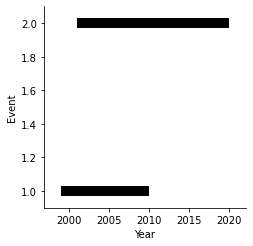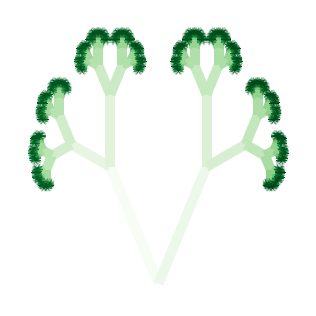# Segments#

## Title: Segments Element#

Dependencies Matplotlib

Backends Bokeh, Matplotlib

```import numpy as np
import holoviews as hv
from holoviews import dim
hv.extension('matplotlib')
````Segments` visualizes a collection of line segments, each starting at a position (`x0`, `y0`) and ending at a position (`x1`, `y1`). To specify it, we hence need four key dimensions, listed in the order (`x0`, `y0`, `x1`, `y1`), and an arbitrary number of value dimensions as attributes to each line segment.

### Basic usage#

Declare mock data:

```event = [1, 2]
data = dict(
start=[1999, 2001],
end=[2010, 2020],
start_event = event,
end_event = event
)
```

Define the `Segments`:

```seg = hv.Segments(data, [hv.Dimension('start', label='Year'),
hv.Dimension('start_event', label='Event'),
'end', 'end_event'])
```

Display and style the Element:

```seg.opts(color='k', linewidth=10)
```### A fractal tree#

```from functools import reduce
def tree(N):
"""
Generates fractal tree up to branch N.
"""
# x0, y0, x1, y1, level
branches = [(0, 0, 0, 1)]
theta = np.pi/5 # branching angle
r = 0.5 # length ratio between successive branches

# Define function to grow successive branches given previous branch and branching angle
angle = lambda b: np.arctan2(b-b, b-b)
length = lambda b: np.sqrt((b-b)**2 + (b-b)**2)
grow = lambda b, ang: (b, b,
b + r*length(b)*np.cos(angle(b)+ang),
b + r*length(b)*np.sin(angle(b)+ang))
ctr = 1
while ctr<=N:
yield branches
ctr += 1
branches = [[grow(b, theta), grow(b, -theta)] for b in branches]
branches = reduce(lambda i, j: i+j, branches)

t = reduce(lambda i, j: i+j, tree(14))
data = np.array(t[1:])
```

Declare a `Segments` Element and add an additional value dimension `c` that we can use for styling:

```s = hv.Segments(np.c_[data, np.arange(len(data))], ['x', 'y', 'x1', 'y1'], 'c')
```

Now, let’s style the Element into a digital broccoli painting:

```s.opts(xaxis=None, yaxis=None, fig_size=150, linewidth=10,
color=np.log10(1+dim('c')), cmap='Greens')
```This web page was generated from a Jupyter notebook and not all interactivity will work on this website. Right click to download and run locally for full Python-backed interactivity.